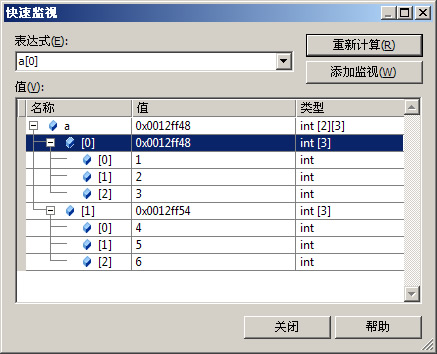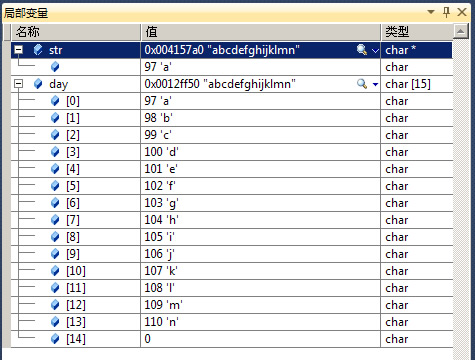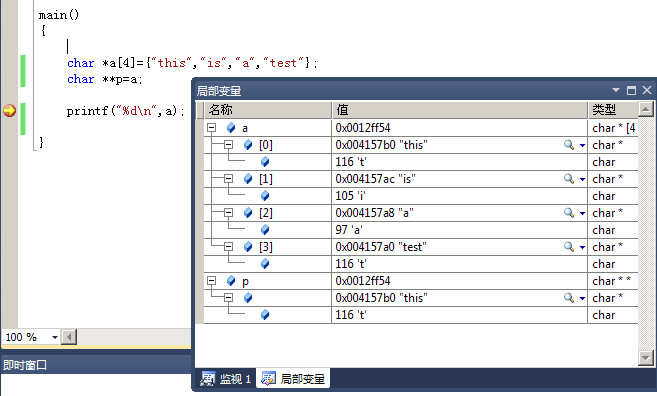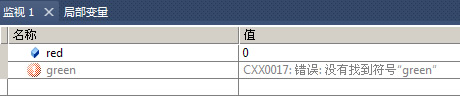# C和指针学习

C和指针学习

1.编译

#cc program.c

gcc编译器的编译自定义格式

#cc -o hello hello.c

#gcc -o hello hello.c

扩充阅读：Linux编译器GCC的使用

http://blog.csdn.net/21aspnet/article/details/1534108

http://blog.csdn.net/21aspnet/article/details/167420

http://blog.csdn.net/21aspnet/article/details/6723758

2.执行

#./a.out

#cc a.c   b.c   c.c

3.产生目标文件

#cc -c program.c

#cc -c program.c  a.c  b.c

4.链接几个目标文件

#cc a.o  b.o  c.o

5.转义字符

\\代表\

\\\\代表\\

\'代表'

\"代表"

\n换行

\r回车

扩充阅读：C语言 格式控制符 和 转义字符

6.注释

7.保留字

8.整型

singed 有符号

`unsigned是无符号的意思，也就是说如果你的编译系统给int分配的存储单元的长度是2个字节的话，有符号的int 取值范围是-32768（即2^15）——32767（即2^15-1），而无符号的unsigned  int就是0-65535（2^16-1）`

 类型 最小范围 char 0-127 signed char -127-127 unsinged char 0-255 int -32767-32767 long int -2147483647-2147483647 unsinged int 0-65536

int a=8;

int a=012;//8进制是0开头

int a=0x0a;//16进制是0x开头

3种输出printf("%d",a);都是10

9.枚举类型

enum A{a,b,c,d};

10.浮点

float double long double

11.指针

int  a;

int *b=&a;

int **c=&b;

a=1;

*b=2;

main()

{

swap(a,b,c)

}

swap(int a,int *b,int **c)

{

a=*b+**c;//这样不能改变值

*b=a+**c;//可以改变值

}

printf("%d",*b);//输出2

printf("%d",a);//输出2

//指针和字符串

char * ch="abc";

char * cp=ch;

printf("%s",cp);//输出abc

//指针和字符

char  ch=‘a’;

char * cp=&ch;

printf("%c",*cp);//输出a

http://blog.csdn.net/21aspnet/article/details/317866

C指针本质

12.typedef

typedef int k;

k k1=10;

int a=0;

typedef int * p;

p b=&a;

13.const常量

const int a=15;//必须声明时赋值

int const a=15;//必须声明时赋值，const在前在后都可以

const int a;

a=15

int ax(const int aa)

{

int a=aa;

return a+1;

}

14.define常量

#defined MMX 50

printf("%d",MMX);

15.链接属性

external外部

internal内部

none无

16.变量类型

auto 变量

static 变量

static int a=5;

external 变量

int a=5;//定义

#include "f.h" //引用，注意不能<"f.h">

extern int a;//声明

printf("%d",a);

static external 变量

register 变量

extern 和static可以函数外

http://blog.csdn.net/21aspnet/article/details/257511

17.语句

if...else...

while循环

i=0;a=0;

while(i<10)

{

a=a+i;

i++;

}

break：退出大循环

continue：退出本次循环

fo循环

for(int i=0;i<2;i++)

{

}

do....while

switch

switch(ch){

case "A":

i+1;

break;

case "B":

i+2;

break;

default:

i=0;

}

goto跳转

goto AA;

AA:if...else

18.操作符

+-*/%

19.位移和位操作符

<<左移

>>右移

&位与

|位或

^位非

20.逻辑运算符

&& ||

21.条件运算符

a>5?b-6:c/2

a大于5就执行b-6否则执行c/2

22.++

K++ ：k不变

++K ：K+1

23.布尔值

C语言中没有布尔类型。任何一个整型的变量都可以充当布尔变量，用0表示False，其它数（默认为1）表示True。

a = 1;
if(a) {printf("a is T\n");}else{printf("a is F\n");}//输出T
a = 2;
if(a) {printf("a is T\n");}else{printf("a is F\n");}//输出T
a = -1;
if(a) {printf("a is T\n");}else{printf("a is F\n");}//输出T
a = 0;
if(a) {printf("a is T\n");}else{printf("a is F\n");}//输出F
if(!a) {printf("a is T\n");}else{printf("a is F\n");}//输出T

#include "stdbool.h"
`bool a = true;if (a) printf("a is true");`

#define False 0

#define True 1

24.数组

int a[]={1,2,3,4,5}

a//用序号输出数组元素，默认下标从0开始

int *ap=a+2;

ap;//输出3

int a[]={1,2,3,4,5,6};//自动计算数组长度

int i;

for(int i;i<6;i++)

{

a[i];这样输出

int *p=a;注意不是&a;

p[i];方法一就是数组元素

*(p+i);方法二

for()

{

printf("%d",*p);//方法三

p++;

int a[]={1,2,3,4,5} ;

int b[]={6,7,8,9,10} ;

int *p;p=b;

int i;

for(i=o;i<10;i++)

{

prinft("d%",*p);

p++;

}

p=a;

for(i=-5;i<5;i++)

{

prinft("d%",*p);

p++;

}

int a={1,2,3,4,5,6};a=1;

a=2;

a=3;

int a={

{1,2,3},

{4,5,6}

};

int a[]={1,2,3,4,5,6};

//声明指针数组，每个指针元素都初始化为指向各个不同的字符串常量

char const keyword[]={

"do";

"doo";

"doooo";

"do";

"doo";

"do";

"doooooo";

}

//声明矩阵，每一维长度都是9，不够用0补齐

char const keyword[]={

"do";

"doo";

"doooo";

"do";

"doo";

"do";

"doooooo";

}

http://blog.csdn.net/21aspnet/article/details/1539928

25.字符串

char day = "abcdefghijklmn";//定义一

char day[] = "abcdefghijklmn";//定义一
char * str="abcdefghijklmn";//定义二

windows下VS2010调试窗口看区别char str="0123456789";//str是编译期大小已经固定的数组
int a=strlen(str); //a=10;//strlen()在运行起确定
int b=sizeof(str); //而b=20;//sizeof()在编译期确定

sizeof 运算符是用来求内存容量字节的大小的。而strlen是用来求字符串实际长度的。

char msg[]={'a','b','c'}

char msg[]={abc}

char *ans;

ans=strchr(day,'c');

printf("%s",ans);//输出从c开始的部分

printf("%d",*ans);//输出找到的指针

ans=strrchr(day,'c');

printf("%s",ans);//输出找到的指针

printf("%d",*ans);//输出找到的指针

char temp="hello";

char *t="world";

strcat(temp,t);

char *a={"this","is","a","test"};
char **p=a;26.结构

struct  simple{

int a;

int b;

};

struct  simple s1;

s1.a=1;

typedef struct {

int a;

int b;

};simple

simple s1;

s1.a=1;

typedef struct {

int a;

int b;

};simple{1,2}

simple.a;//输出1

struct data {

int a;

int b;

};data1{1,2}

struct data *p;

p=&data1;

(*p).a;//输出1

p->b;//输出2

27.内联函数

inline int 函数名(参数)

inline int 函数名(参数)

{

}

28.宏

#define SQUARE(x)   x*x

29.函数

int a=1;

int b=2;

ext1(a,b);

ext1(int x.int y)

{

int temp=x;

x=y;

y=temp;

prinft("x=%d,y=%d",x,y)

}

30.动态内存分配

#include <malloc.h>//malloc
#include <stdlib.h>//exit’
int * p;

p=malloc(100);

if(p==NULL)

{

printf("Out of memory!\n");

exit(1);

}

http://blog.csdn.net/21aspnet/article/details/146968

31.链表

//先定义一个结构体

typedef struct node{

int data;//数据域

struct node * next;//指针域  ，是struct node的成员，又指向struct node类型的的数据，这里存放下个结点的地址

}Node;

//返回类型是之前定义的结构体

Node * creat()

//需要先定义3个节点

Node *p=NULL;//指向原链表的最后一个结点

Node *s=NULL;//指向新节点

//用while循环

int c=1;//为0是退出循环的条件

int d=0;

while(c)

{

//使用scanf接受用户数据数据

scanf("%d",&d);

if (d!=0)//继续输入

{

s->data=d;//给新节点赋值

p->next=s;//将第一个结点链接在第二个结点后面

p=s;//p继续指向当前节点

}

else

{

c=0;//用户输入0结束 输入

}

}

p->next=NULL;//结束输入时将最后结点定义为尾结点

}

//输出结点

{

//新定义一个指针用于遍历

while(p!=NULL)

{

printf("%d",p->data);

p=p->next;//注意while循环里一定要让P指针不断往下指向

}

}

//查找制定元素的顺序

{

int t=0;

printf("请输入您要查找的值：\n");

scanf("%d",&t);

int k=0;

while(p!=NULL)

{

k++;

if(p->data==t)

{

printf("%d是第%d个元素：\n",t,k);

break;

}

p=p->next;

}

if(p==NULL)

{

printf("没找到");

}

}

//元素之间插入值

{

Node *s=NULL;//指向新节点

s=(Node*)malloc(sizeof(Node));//动态分配内存空间

int t=0;

int i=0;

printf("请输入您要查找的值：\n");

scanf("%d",&t);

printf("请输入您要插在那个元素后面：\n");

scanf("%d",&i);

while(p!=NULL)

{

if(p->data==i)

{

s->data=t;

s->next=p->next;//注意是先指向新节点，再改旧节点

p-next=s;

printf("插入成功：\n");

break;

}

p=p->next;

}

if(p==NULL)

{

printf("没找到\n");

}

}

32.scanf

int d=0;

注意是&d而不是d，因为是改变变量的地址对应的内容，而不是直接取其值

33.union

struct可以定义一个包含多个不同变量的类型，每一个变量在内存中占有自己独立的内存空间，可以同时存储不同类型的数据。
uniion也可以定义一个包含多个不同变量类型，但这些变量只共有同一个内存空间，每次只能使用其中的一种变量存储数据。

union{
int   a;
float   b;
char     c;
}a;
a.b=10.123;  printf("%d",a.a);

34.register寄存器

register int a=1;

35.enum枚举

```enum color
{
red,
green=2,
blue=4
}colorVal;

printf("%d\n",red);	```36.条件编译

#ifdef

#else

#endif

#ifndef

#else

#endif

#if

#else

#endif

选择功能是否加入，在编译阶段。

#define DEBUG//定义可调试 不定义则不

#ifnedf DEBUG

#ifdef

#ifnedf FILE_H_

#define FILE_H_

#define     定义宏
#undef      取消已定义的宏
#if         如果给定条件为真，则编译下面代码
#ifdef      如果宏已经定义，则编译下面代码
#ifndef     如果宏没有定义，则编译下面代码
#elif       如果前面的#if给定条件不为真，当前条件为真，则编译下面代码
#endif      结束一个#if……#else条件编译块
#error      停止编译并显示错误信息

37. size_t

size_t一般用来表示一种计数，比如有多少东西被拷贝等